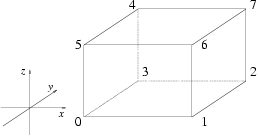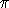## CGAL::Iso_cuboid_3<Kernel>

### Definition

An object s of the data type Iso_cuboid_3<Kernel> is a cuboid in the Euclidean space3 with edges parallel to the x, y and z axis of the coordinate system.

Although they are represented in a canonical form by only two vertices, namely the lexicographically smallest and largest vertex with respect to Cartesian xyz coordinates, we provide functions for ``accessing'' the other vertices as well.

Iso-oriented cuboids and bounding boxes are quite similar. The difference however is that bounding boxes have always double coordinates, whereas the coordinate type of an iso-oriented cuboid is chosen by the user.

### Creation

Iso_cuboid_3<Kernel> c ( Point_3<Kernel> p, Point_3<Kernel> q);
introduces an iso-oriented cuboid c with diagonal opposite vertices p and q. Note that the object is brought in the canonical form.

Iso_cuboid_3<Kernel> c ( Point_3<Kernel> p, Point_3<Kernel> q, int);
introduces an iso-oriented cuboid c with diagonal opposite vertices p and q. The int argument value is only used to distinguish the two overloaded functions.
 Precondition: p.x()<=q.x(), p.y()<=q.y() and p.z()<=q.z().

 Iso_cuboid_3 c ( Point_3 left, Point_3 right, Point_3 bottom, Point_3 top, Point_3 far, Point_3 close);
introduces an iso-oriented cuboid c whose minimal x coordinate is the one of left, the maximal x coordinate is the one of right, the minimal y coordinate is the one of bottom, the maximal y coordinate is the one of top, the minimal z coordinate is the one of far, the maximal z coordinate is the one of close.

 Iso_cuboid_3 c ( Kernel::RT min_hx, Kernel::RT min_hy, Kernel::RT min_hz, Kernel::RT max_hx, Kernel::RT max_hy, Kernel::RT max_hz, Kernel::RT hw = RT(1));
introduces an iso-oriented cuboid c with diagonal opposite vertices (min_hx/hw, min_hy/hw, min_hz/hw) and (max_hx/hw, max_hy/hw, max_hz/hw).
 Precondition: hw0.

### Operations

 bool c.operator== ( c2) Test for equality: two iso-oriented cuboid are equal, iff their lower left and their upper right vertices are equal. bool c.operator!= ( c2) Test for inequality. Point_3 c.vertex ( int i) returns the i'th vertex modulo 8 of c. starting with the lower left vertex. Point_3 c.operator[] ( int i) returns vertex(i), as indicated in the figure below:Point_3<Kernel> c.min () returns the smallest vertex of c (= vertex(0)).

Point_3<Kernel> c.max () returns the largest vertex of c (= vertex(7)).

Kernel::FT c.xmin () returns smallest Cartesian x-coordinate in c.
Kernel::FT c.ymin () returns smallest Cartesian y-coordinate in c.
Kernel::FT c.zmin () returns smallest Cartesian z-coordinate in c.
Kernel::FT c.xmax () returns largest Cartesian x-coordinate in c.
Kernel::FT c.ymax () returns largest Cartesian y-coordinate in c.
Kernel::FT c.zmax () returns largest Cartesian z-coordinate in c.

Kernel::FT c.min_coord ( int i) returns i-th Cartesian coordinate of the smallest vertex of c.
 Precondition: 0i2.

Kernel::FT c.max_coord ( int i) returns i-th Cartesian coordinate of the largest vertex of c.
 Precondition: 0i2.

### Predicates

 bool c.is_degenerate () c is degenerate, if all vertices are collinear. Bounded_side c.bounded_side ( Point_3 p) returns either ON_UNBOUNDED_SIDE, ON_BOUNDED_SIDE, or the constant ON_BOUNDARY, depending on where point p is. bool c.has_on_boundary ( Point_3 p) bool c.has_on_bounded_side ( Point_3 p) bool c.has_on_unbounded_side ( Point_3 p)

### Miscellaneous

Kernel::FT c.volume () returns the volume of c.

Bbox c.bbox () returns a bounding box containing c.

Iso_cuboid_3<Kernel> c.transform ( Aff_transformation_3<Kernel> t)
returns the iso-oriented cuboid obtained by applying t on the smallest and the largest of c.
 Precondition: The angle at a rotation must be a multiple of/2, otherwise the resulting cuboid does not have the same size. Note that rotating about an arbitrary angle can even result in a degenerate iso-oriented cuboid.